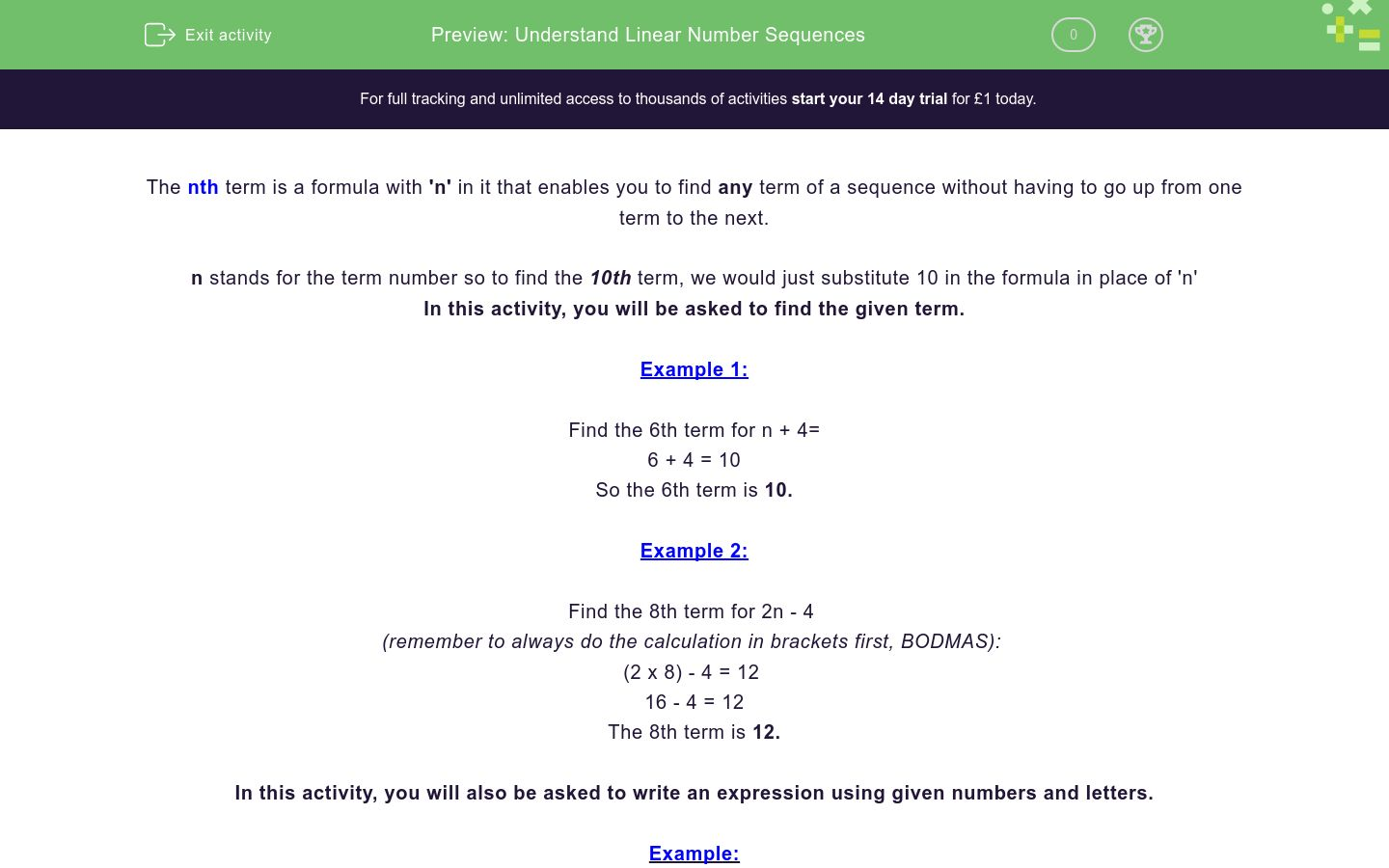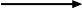# Understand Linear Number Sequences

In this worksheet, students will be tested on their understanding of linear number sequences by finding the given terms of different sequences and creating expressions that represent linear number sequences.Key stage:  KS 2

Curriculum topic:   Algebra

Curriculum subtopic:   Know Linear Number Sequences

Difficulty level:### QUESTION 1 of 10

The nth term is a formula with 'n' in it that enables you to find any term of a sequence without having to go up from one term to the next.

n stands for the term number so to find the 10th term, we would just substitute 10 in the formula in place of 'n'

In this activity, you will be asked to find the given term.

Example 1:

Find the 6th term for n + 4=

6 + 4 = 10

So the 6th term is 10.

Example 2:

Find the 8th term for 2n - 4

(remember to always do the calculation in brackets first, BODMAS):

(2 x 8) - 4 = 12

16 - 4 = 12

The 8th term is 12.

In this activity, you will also be asked to write an expression using given numbers and letters.

Example:

The amount received by 6 children when a prize is shared equally between them.

Use the bold type letters and numbers to help:

We would create the expression:

a = p÷6

Now, over to you!

Can you write down the next term of the sequence whose nth term is 8n - 5?

Can you write the next 2 terms in the sequence whose nth term is 9n?

Can you correctly identify the first three terms of a sequence whose nth term is 6n - 5?

1, 6, 12

1, 7, 13

1, 8, 14

Can you write the expression for the length (l) of the lorry?b                               4

Can you choose the two correct expressions for the length (l) of the lorry?12                            f

12 + f

f12

f + 12

12f

Choose the correct expression that will represent the following statement.

There are c cars in a car park and then 5 more arrive.c5

c - 5

c + 5

c / 5

Can you match the following statements with the correct expression?

1. There are s sweets in a box, then someone eats 6 of them.

2. Tony had 15 stickers. He gave 'g' stickers to his friends. How many stickers does he have left?

## Column B

There are s sweets in a box, then someone eats 6 o...
(5+n) ÷ 3
Tony had 15 stickers. He gave 'g' stickers to his ...
s - 6
15 - g

Which one of the following word expressions fails to adequately express the following statement? (Use the letters and numbers in bold to help you.)

The total money raised in a sponsored swim at £3 for each length.

t = 3l

l = 3t

Can you find the expressions for the following statement?

2 x 5 x n x 2

(5xn)²

(5n)²

2 x - n x 5

The formula for the length left on a 40 m roll of carpet is

R = 40 - C

Use this formula to work out R for the two questions below and choose the correct answers:

a. C = 12

b. C = 19• Question 1

Can you write down the next term of the sequence whose nth term is 8n - 5?

3
EDDIE SAYS
Don't forget, n stands for the term number so to find the 8th term, we would just substitute 8 in the formula in place of 'n'. 8n - 5 = 8 x 1 = 8 - 5 = 3
• Question 2

Can you write the next 2 terms in the sequence whose nth term is 9n?

9
18
EDDIE SAYS
The same rule applies. 'n' stands for the term number so to find the 9th term, we would just substitute 9 in the formula in place of 'n'. 9 x 1 = 9 9 x 2 = 18 1st term = 9 2nd term = 18 Got it? No worries if not, let's keep practising.
• Question 3

Can you correctly identify the first three terms of a sequence whose nth term is 6n - 5?

1, 7, 13
EDDIE SAYS
Did you get it? There were a tricky range of options close to the correct answer! Again, don't forget 'n' stands for the term number so, in order to find the 6th term, we would just substitute 6 in the formula in place of 'n'. 6 x 1 = 6 - 5 = 1 6 x 2 =12 - 5 = 7 6 x 3 = 18 - 5 = 13 1st term = 1 2nd term = 7 3rd term = 13
• Question 4

Can you write the expression for the length (l) of the lorry?b                               4

b + 4
4 + b
EDDIE SAYS
We can write the expression for the length of the lorry in two ways, either b + 4 or 4 + b both will equal l (length). Simple.
• Question 5

Can you choose the two correct expressions for the length (l) of the lorry?12                            f

12 + f
f + 12
EDDIE SAYS
We can write the expression for the length of the lorry in two ways, either 12 + f or, f + 12, both will equal l (length).
• Question 6

Choose the correct expression that will represent the following statement.

There are c cars in a car park and then 5 more arrive.c + 5
EDDIE SAYS
For this worded problem, the expression is c + 5. c represents the cars that are already parked in the car park and + 5 shows that 5 extra cars have arrived. You're getting better at this with every attempt!
• Question 7

Can you match the following statements with the correct expression?

1. There are s sweets in a box, then someone eats 6 of them.

2. Tony had 15 stickers. He gave 'g' stickers to his friends. How many stickers does he have left?

## Column B

There are s sweets in a box, then...
s - 6
Tony had 15 stickers. He gave 'g'...
15 - g
(5+n) ÷ 3
EDDIE SAYS
We had different types of calculations to use to create the correct expressions. 1. There are s sweets in a box, then someone eats 6 of them. s - 6 2. Tony had 15 stickers. He gave 'g' stickers to his friends. How many stickers does he have left? 15 - g 3. Add 5 to n and then divide your answer by 3 = (5 + n) ÷ 3 For this last question, remember BODMAS, it tells which order to do things in. BODMAS = Brackets, Orders, Division, Multiplication, Addition, Subtraction.
• Question 8

Which one of the following word expressions fails to adequately express the following statement? (Use the letters and numbers in bold to help you.)

The total money raised in a sponsored swim at £3 for each length.

t = 3l
EDDIE SAYS
That made us think! So the short rule can be written in two possible different ways: t = 3 x l t = 3l
• Question 9

Can you find the expressions for the following statement?

(5xn)²
(5n)²
EDDIE SAYS
As before, we were told the order to complete the equation so we have to use brackets to show this. (5xn)² = (5n)² Remember BODMAS, it tells which order to do things in. BODMAS = Brackets, Orders, Division, Multiplication, Addition, Subtraction.
• Question 10

The formula for the length left on a 40 m roll of carpet is

R = 40 - C

Use this formula to work out R for the two questions below and choose the correct answers:

a. C = 12

b. C = 19EDDIE SAYS
Here we had to use our knowledge of the expression of R = 40 - c to find the correct answer. a. R = 40- 12 = 28 b. R = 40 - 19 = 21 Well done! That's another activity completed. Why not have an attempt at another so you feel really confident?
---- OR ----

Sign up for a £1 trial so you can track and measure your child's progress on this activity.

### What is EdPlace?

We're your National Curriculum aligned online education content provider helping each child succeed in English, maths and science from year 1 to GCSE. With an EdPlace account you’ll be able to track and measure progress, helping each child achieve their best. We build confidence and attainment by personalising each child’s learning at a level that suits them.

Get started